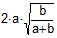Problem 278: Tangent Circles, Common External Tangent, Chord Level: High School, SAT Prep, College geometry. In the figure below, the circles of centers A and B with radius a and b, respectively, are tangent externally at point C. If DE is the common external tangent, prove that chord CD =.Recent Additions